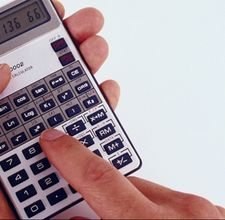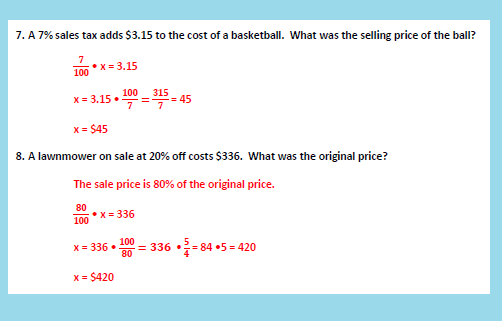# How to Do Percentages

If you are to calculate marks out of 100 it is usually not difficult so as to find the percentage. Nevertheless, if the marks you have obtained are not exactly out of 100 and you are calculating percentage, it may prove to be quite confusing and problematic for some students as well as for some elderly people. When we have to make daily life calculations, the total is not always hundred. It is worth-mentioning here that the percentages are always calculated out of 100% irrespective of the fact how long is the figure we are trying to find the percentage for. This only involves a few things that you should remember while solving percentages.

### Instructions

• 1

First of all you need you need to know two figures. One should be the total amount out of which you are finding the percentage and the mere amount the percentage of which you have to find.

• 2

If the total amount is other than hundred then you have to first put this in fraction form. This is done by placing the total or the larger amount in the denominator and the smaller amount or the one for which you have to calculate the percentage in the numerator.

• 3

Once you have put the two amounts in the fraction form, make the calculation manually or by using the calculator if the working is a complex one.• 4

As you will calculate, the figure you will get will ofcourse be in decimals. Now you will multiply the figure with 100 and you will get the percentage. For example:

135/150 = 0.9
0.9 x 100 = 90%

• 5

Now if you are to find the amount against a certain given percentage such as 17% of 150 then you have to simple have to multiply 17% with the total amount in the following way:

17% x 150
17/100 x 150 = 25.5

• 6

In the above step, make sure that when you are finding the amount instead of percentage, like you have to find your marks in figures from the percentage, simply divide the percentage with 100 and multiply it with the total amount.•